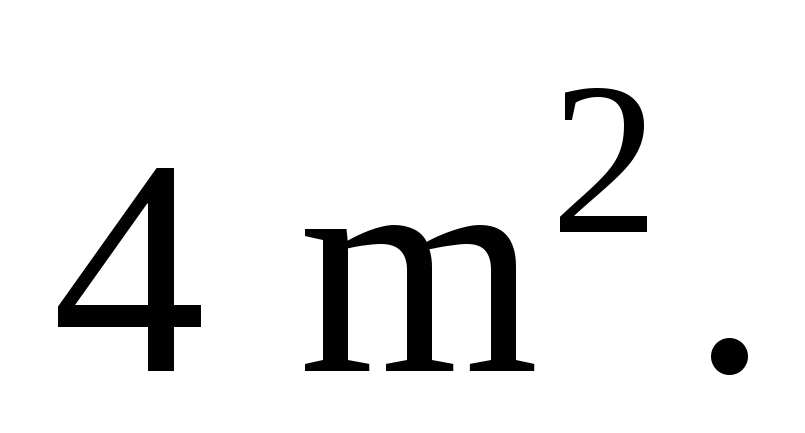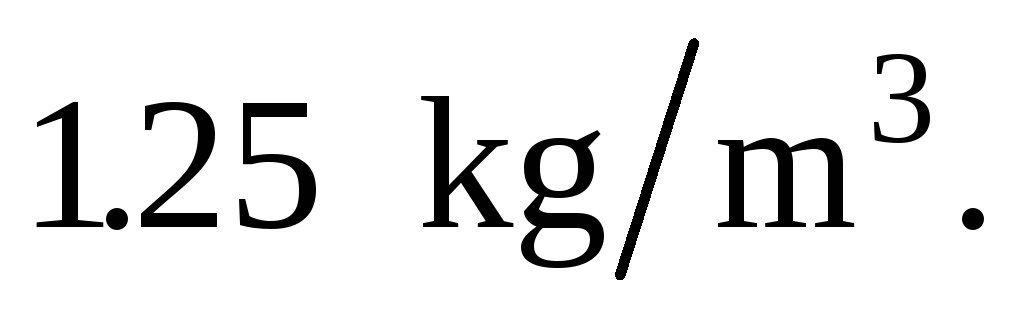# Drag Force and Terminal Velocity Problems Fishbane Chp 5

 Drag Force and Terminal Velocity Problems Fishbane Chp 5 Q33. Consider Eq. (5–32) for the drag force, which has two terms. Which term, if any, dominates at low speeds and which, if any, dominates at high speeds? How would you decide the size of the domains for which one term is much larger than the other? The first term is proportional to v while the second one is proportional to v2; so at low speeds the first term dominates (as v is small and v2 is even smaller), while at high speeds the second term dominates (as v is large but v2 is even larger). At a certain speed, v1, the two terms become the same: bv1 = cv12, or v1 = b/c. When v << v1 it’s the low-speed regime, where the first term dominates; and when v >> v1 it’s the high-speed regime, where the second one dominates. P37. Estimate the drag force on an automobile cruising atAssume that the drag coefficientis 0.45 and that the car’s cross-sectional area isTake air to have a density ofDownload 459.96 Kb.Share with your friends: